Apertium has moved from SourceForge to GitHub.
If you have any questions, please come and talk to us on #apertium on irc.freenode.net or contact the GitHub migration team.

# Part-of-speech tagging

(Redirected from Apertium-tagger)

## Contents

Part-of-speech tagging is the process of assigning unambiguous grammatical categories to words in context. The crux of the problem is that surface forms of words can often be assigned more than one part-of-speech by morphological analysis. For example in English, the word "trap" can be both a singular noun ("It's a trap!") or a verb ("I'll trap it").

This page intends to give an overview of how part-of-speech tagging works in Apertium, primarily within the apertium-tagger, but giving a short overview of constraints (as in constraint grammar) and restrictions (as in apertium-tagger) as well.

Some projects refer to both analysis and disambiguation as "tagging" – in apertium, it refers only to morphological disambiguation.

## Introduction

Consider the following sentence in Spanish ("She came to the beach"):

Vino (noun or verb) a (pr) la (det or prn) playa (noun)

We can see that two out of the four words are ambiguous, "vino", which can be a noun ("wine") or verb ("came") and "la", which can be a determiner ("the") or a pronoun ("her" or "it"). This gives the following possibilities for the disambiguated analysis of the sentence:

Tagset
Tag Gloss
det Determiner
noun Noun
prn Pronoun
pr Preposition
verb Verb
noun, pr, det, noun → Wine to the beach
verb, pr, det, noun → She came to the beach
noun, pr, prn, noun → Wine to it beach
verb, pr, prn, noun → She came to it beach

As can be seen, only one of these interpretations (verb, pr, det, noun) yields the correct translation. So the task of part-of-speech tagging is to select the correct interpretation. There are a number of ways of doing this, involving both linguistically motivated rules (as constraint grammar and the Brill tagger) and statistically based (such as the TnT tagger or the ACOPOST tagger).

The tagger in Apertium (apertium-tagger) uses a probabilistic (hidden Markov) model, which is statistically trained, but can be influenced by "rules".

## Preliminaries

Before we explain what a hidden Markov model is, we need to give some preliminaries, that is define what we mean by tagset and ambiguity class. The tagset (often shown as Γ) is the set of valid tags (parts of speech, etc.) to be used in the model, for example: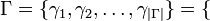$\Gamma = \{ \gamma_1, \gamma_2, \ldots, \gamma_{|\Gamma|} \} = \{$ '<noun>', '<verb>', '<adj>',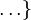$\ldots \}~$
Ambiguity
classes
det/prn/verb
det/prn
noun/verb
det
noun
prn
pr
verb

The ambiguity classes (noted as Σ) of a model are the set of possible ambiguities, for example between noun and verb, or verb and adjective, e.g.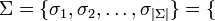$\Sigma = \{ \sigma_1, \sigma_2, \ldots, \sigma_{|\Sigma|} \} = \{$ 'noun|verb', 'det|prn', 'det|prn|verb',$\ldots \}~$

## Hidden Markov models

A hidden Markov model is made up of two matrices, representing transition and emission probabilities and a vector representing the initial probabilities of the model. The is often expressed as: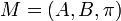$M = (A, B, \pi)~$

Where M is the model, A is the matrix of transition probabilities, B is the matrix of emission probabilities and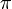$~\pi$ is the vector of initial probabilities. These probabilities are calculated between the tag set and the ambiguity classes from a training set. This is referred to as parameter estimation.

The transition probabilities represent the probability of one tag following another, ...

## Parameter estimation

### Maximum likelihood

The easiest way to estimate the parameters of a hidden Markov model is to use maximum likelihood (ML). This method requires a pre-tagged corpus. We're going to make a very small training corpus so that we can train a model which can be used to disambiguate the example sentence above. The corpus is much smaller than would be normally used, but will let us demonstrate step-by-step how the model is constructed and used.

Untagged Analysed Tagged
Vino a la playa Vino<noun>/<verb> a<pr> la<det>/<prn> playa<noun> .<sent> Vino<verb> a<pr> la<det> playa<noun> .<sent>
Voy a la casa Voy<verb> a<pr> la<det>/<prn> casa<noun>/<verb> .<sent> Voy<verb> a<pr> la<det> casa<noun> .<sent>
Bebe vino en casa Bebe<verb> vino<noun>/<verb> en<pr> casa<noun>/<verb> .<sent> Bebe<verb> vino<noun> en<pr> casa<noun> .<sent>
La casa es grande La<det>/<prn> casa<noun>/<verb> es<verb> grande<adj> .<sent> La<det> casa<noun> es<verb> grande<adj> .<sent>
Es una ciudad grande Es<verb> una<det>/<prn>/<verb> ciudad<noun> grande<adj> .<sent> Es<verb> una<det> ciudad<noun> grande<adj> .<sent>

In this corpus, the "untagged" text would come from anywhere, the "analysed" text would be the result after being passed through a morphological analyser, and the "tagged" text would be manually disambiguated from the analysed text by one or more humans.

Matrix of transition counts
Second tag
First tag verb noun det prn pr adj sent
verb 0 1 1 0 2 1 0
noun 1 0 0 0 1 1 3
det 0 4 0 0 0 0 0
prn 0 0 0 0 0 0 0
pr 0 1 2 0 0 0 0
adj 0 0 0 0 0 0 2
sent 3 0 1 0 0 0 0
Calculating the transition probabilities

The transition probabilities are basically the set of probabilities that a certain tag follows a certain other tag. We're training a bigram model, so we need to make a two-dimensional matrix. The axes of this matrix are the tag set. The first thing we do is sum up the transition counts. For example, if we see a determiner, then a noun four times in the course of the tagged corpus, then we put a four in the element (det, noun).

It should be possible to see from the counts in the table on the right that already this models some information about the corpus we have. We can see that determiners are followed by nouns and that verbs very often start a sentence. If the corpus were larger, there would be fewer zeroes. These counts are quite easy to calculate.

The next thing to do is calculate the matrix of transition probabilities, A. This is usually written as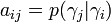$a_{ij} = p(\gamma_j | \gamma_i)~$

and means the element at aij is the probability of γj appearing given the previous tag γi. We can give the example for determiner followed by noun as: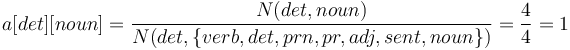$a[det][noun] = \frac{N(det, noun)}{N(det, \{verb,det,prn,pr,adj,sent,noun\})} = \frac{4}{4} = 1$
Matrix of transition probabilities (A)
Second tag (γj)
First tag (γi) verb noun det prn pr adj sent
verb 0 0.2 0.2 0 0.4 0.2 0
noun 0.16 0 0 0 0.16 0.16 0.5
det 0 1 0 0 0 0 0
prn 0 0 0 0 0 0 0
pr 0 0.3 0.6 0 0 0 0
adj 0 0 0 0 0 0 1
sent 0.75 0 0.25 0 0 0 0

Or for a more ambiguous example:$a[pr][noun] = \frac{N(pr, noun)}{N(pr, \{verb,det,prn,pr,adj,sent,noun\})} = \frac{1}{3} \approx 0.33$

Where N(x) stands for the number of time that x is seen in the training set.

Calculating the emission probabilities

We follow a similar process for calculating the emission probabilities of the model, only this time, we're counting the number of times that an ambiguity class is seen in the untagged corpus given a tag in the tagged corpus over the number of times all the other ambiguity classes have been seen. This is expressed as: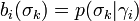$b_i(\sigma_k) = p(\sigma_k|\gamma_i)~$

Here, σk is the given ambiguity class, and pk | γi) is the probability of seeing the the ambiguity class σk given the tag γi. So, to continue the example, in the above tagged corpus we see the tag "verb" five times, and the ambiguity class "verb|noun" once.

Matrix of emission probabilities (B)
Second tag (γj)
First tag (σk) verb noun det prn pr adj sent
det/prn/verb 0 0 0.25 0 0 0 0
det/prn 0 0 0.75 0 0 0 0
noun/verb 0.2 0.67 0 0 0 0 0
verb 1 0 0 0 0 0 0
noun 0 1 0 0 0 0 0
det 0 0 0 0 0 0 0
prn 0 0 0 0 0 0 0
pr 0 0 0 0 1 0 0
adj 0 0 0 0 0 1 0
sent 0 0 0 0 0 0 1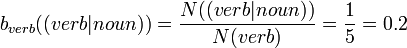$b_{verb}((verb|noun)) = \frac{N((verb|noun))}{N(verb)} = \frac{1}{5} = 0.2$

And given the tag "noun" which we see six times, we see the ambiguity class "verb|noun" four times,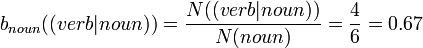$b_{noun}((verb|noun)) = \frac{N((verb|noun))}{N(noun)} = \frac{4}{6} = 0.67$
Calculating the initial probabilities

The vector for the initial probabilities of the model is just the row from the transition probability matrix which corresponds to the end of sentence marker tag <sent> (in the equations marked as #), that is: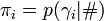$\pi_i = p(\gamma_i|\#)$

So given our above data set: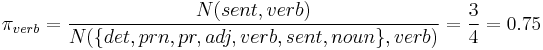$\pi_{verb} = \frac{N(sent, verb)}{N(\{det,prn,pr,adj,verb,sent,noun\}, verb)} = \frac{3}{4} = 0.75$

## Tagging

So, we've got the model set up, that we hope describes the text. The next thing to do is use it. Let's say we have the ambiguous example sentence we gave above:

Vino a la playa

We want to disambiguate this using the model we've trained above. In order to do this, we use the Viterbi algorithm, as will be explained below.

### Viterbi

The Viterbi algorithm is then used to tag the sentence. Basically, it consists in an efficient way to compute the most likely path through a sequence of probabilities (POS-tags for each words, bigrams, trigrams).